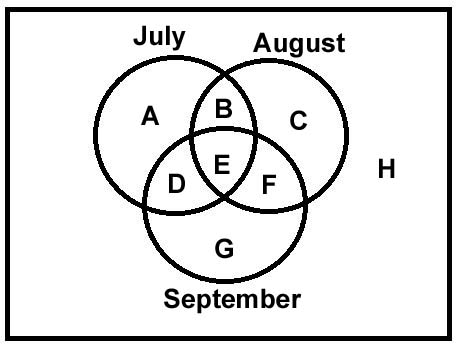Question 13

# A survey was conducted of 100 people to find out whether they had read recent issues of Golmal, a monthly magazine. The summarized information regarding readership in 3 months is given below:Only September: 18;September but not August: 23;September and July: 8;September:28;July: 48;July and August: 10;none of the three months: 24What is the number of surveyed people who have read exactly two consecutive issues (out of the three)?

SolutionLet the areas be labelled as shown in the diagram above.

The number of people corresponding to "none of the three months" is 24. So, H is 24.

Only September is 18. So, G = 18

September but not August is 23. So, G + D = 23.

Hence, D = 23 - 18 = 5.

We know that September and July is 8. So, D + E = 8

This implies E = 3.

September = 28. So, D + E + F + G = 28.

So, F = 28 - 5 - 3 - 18 = 2.

July and August = 10.

So, B + E = 10.

E = 3. So, B = 7.

July = 48.

So, A + B + D + E = 48

A = 48 - 7 - 5 - 3 = 33.

There are 100 people in total. So, C = 100 - A - B - D - E - F - G - H = 100 - 33 - 7 - 5 - 3 - 2 - 18 - 24 = 8

So, number of people who read exactly two consecutive issues = (July & August) + (August & September) = B + F = 7 + 2 = 9